1597062900

# The Intuitive Explanation of Logistic Regression

## Introduction

Logistic regression can be pretty difficult to understand! As such I’ve put together a very intuitive explanation of the why, what, and how of logistic regression. We’ll start with some building blocks that should lend well to clearer understanding so hang in there! Through the course of the post, I hope to send you on your way to understanding, building, and interpreting logistic regression models. Enjoy!

## What is Logistic Regression?

Logistic regression is a very popular approach to predicting or understanding a binary variable (hot or cold, big or small, this one or that one — you get the idea). Logistic regression falls into the machine learning category of classification.

One more example for you to distinguish between linear and logistic regression: Rather than predicting how much something will be sold for… you alternatively are predicting whether it will be sold or not. Without further adieu, let’s dive right in!

## Understanding Linear Regression as a Precursor

Let’s talk about the output of a linear regression. For those of you who aren’t familiar with linear regression it would be best to start there. You can visit this post to learn about Simple Linear Regression & this one for Multiple Linear Regression.

Now knowing a bit about linear regression; you’d know that the linear regression output is equatable to the equation of a line. Simply enough… that’s all we want; just a way to reinterpret one variable to lend insight into another.

So knowing this, let’s run a linear regression on a binary variable. Binary being yes or no, 1 or 0. This variable will be the candidate for our logistic regression, but we’ll get there shortly.

## Building a Linear Regression to Understand Logistic Regression

Today we’ll work with the `mtcars` dataset. This is a classic dataset for data science learning that details fuel consumption, engine details, among other details for a variety of automobiles.

Quick glimpse at the dataset:

``````glimpse(mtcars)
``````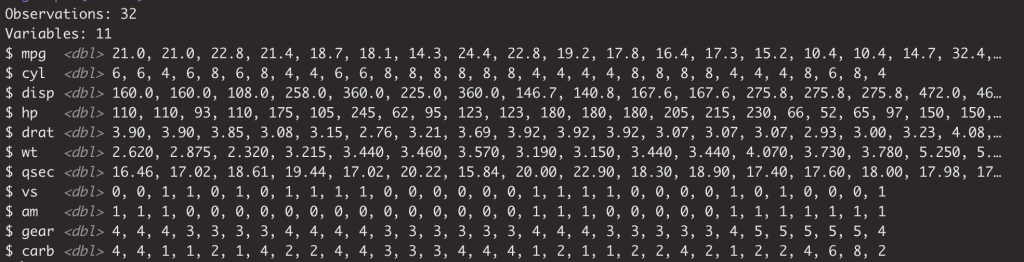``````head(mtcars)
``````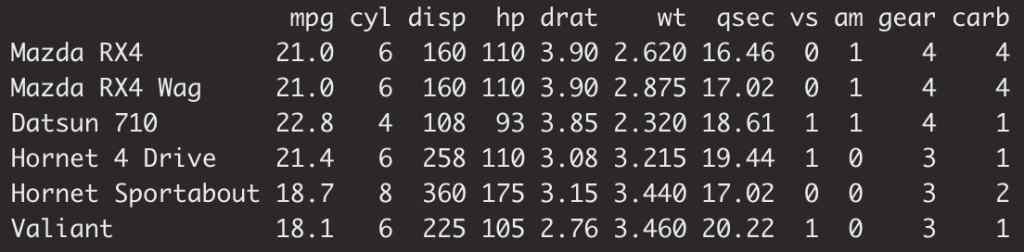## Linear Regression for a Binary Variable

In this dataset, we have one binary variable… `vs`. Not knowing much about cars, I won’t be able to give you a detailed explanation of what `vs` means, but the high level is it’s representative of the engine configuration… I also know that the configuration has impact on things like power, efficiency, etc. which is something we’d be able to tease out through our models. So hopefully it will be easy to determine the difference!

Let’s build that regression model! We’ll seek to understand `vs` as a function of miles per gallon.

#software-engineering #statistics #data-science #machine-learning #software-development #deep learning

## Buddha Community1598352300

## Regression: Linear Regression

Machine learning algorithms are not your regular algorithms that we may be used to because they are often described by a combination of some complex statistics and mathematics. Since it is very important to understand the background of any algorithm you want to implement, this could pose a challenge to people with a non-mathematical background as the maths can sap your motivation by slowing you down.In this article, we would be discussing linear and logistic regression and some regression techniques assuming we all have heard or even learnt about the Linear model in Mathematics class at high school. Hopefully, at the end of the article, the concept would be clearer.

**Regression Analysis **is a statistical process for estimating the relationships between the dependent variables (say Y) and one or more independent variables or predictors (X). It explains the changes in the dependent variables with respect to changes in select predictors. Some major uses for regression analysis are in determining the strength of predictors, forecasting an effect, and trend forecasting. It finds the significant relationship between variables and the impact of predictors on dependent variables. In regression, we fit a curve/line (regression/best fit line) to the data points, such that the differences between the distances of data points from the curve/line are minimized.

#regression #machine-learning #beginner #logistic-regression #linear-regression #deep learning1592023980

## 5 Regression algorithms: Explanation & Implementation in Python

Take your current understanding and skills on machine learning algorithms to the next level with this article. What is regression analysis in simple words? How is it applied in practice for real-world problems? And what is the possible snippet of codes in Python you can use for implementation regression algorithms for various objectives? Let’s forget about boring learning stuff and talk about science and the way it works.

#linear-regression-python #linear-regression #multivariate-regression #regression #python-programming1597407900

## Logistic Regression : Intuition of Logistic Regression

Prerequisite: This assumed that you understand the concept of supervised algorithm and preliminary difference between classification and regression. Otherwise you can refer here.

In the world of Machine Learning, beginners finds it quite challenging to understand Logistic Regression both for it term and similarity & difference with Linear Regression. This algorithm is very popular classification algorithm.

To understand it further, it is also recommended to have an intuitive knowledge on Simple Linear Regression. For that, you can refer here.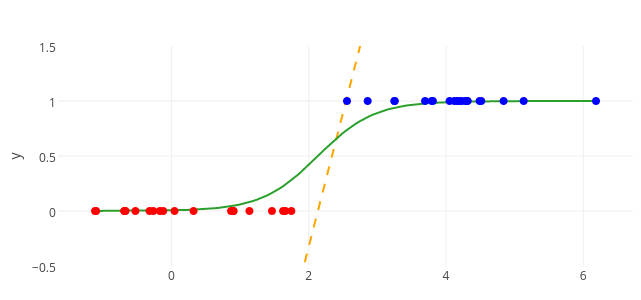Linear Regression and Logistic Regression

## Intuition of Logistic Regression

Linear Regression helps us to predict stock prices, employee’s salary or temperature of a day etc, so mainly helps in prediction of continuous variable. Whereas, Logistic Regression deals with other type of problem like spam detection, employee retention, customer identification etc, hence, this help in prediction of categorical variable. The later technique is known as classification, more specifically Binary Classification.

Now, to understand the Logistic Regression, let’s consider the following example.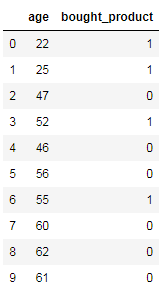Figure 1. Sample Datasets

Here, we need to find out potential customers of smartphones based on the age.

In this example, you can observe a pattern like younger customer is more likely to buy a smartphone (say, 1) whereas old customers doesn’t invest much money on it (say, 0). So, understanding correlation we can try and build a machine learning model for the same.

#machine-learning #logistic-regression #towards-data-science #sigmoid-curves #deep learning1600123860

## Linear Regression VS Logistic Regression (MACHINE LEARNING)

Linear Regression and Logistic Regression are** two algorithms of machine learning **and these are mostly used in the data science field.

Linear Regression:> It is one of the algorithms of machine learning which is used as a technique to solve various use cases in the data science field. It is generally used in the case of continuous output. For e.g if ‘Area’ and ‘Bhk’ of the house is given as an input and we have found the ‘Price’ of the house, so this is called a regression problem.

Mechanism:> In the diagram below X is input and Y is output value.

#machine-learning #logistic-regression #artificial-intelligence #linear-regression1597019820

## Introduction:

In this article, I will be explaining how to use the concept of regression, in specific logistic regression to the problems involving classification. Classification problems are everywhere around us, the classic ones would include mail classification, weather classification, etc. All these data, if needed can be used to train a Logistic regression model to predict the class of any future example.

## Context:

1. Introduction to classification problems.
2. Logistic regression and all its properties such as hypothesis, decision boundary, cost, cost function, gradient descent, and its necessary analysis.
3. Developing a logistic regression model from scratch using python, pandas, matplotlib, and seaborn and training it on the Breast cancer dataset.
4. Training an in-built Logistic regression model from sklearn using the Breast cancer dataset to verify the previous model.

## Introduction to classification problems:

Classification problems can be explained based on the Breast Cancer dataset where there are two types of tumors (Benign and Malignant). It can be represented as: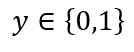where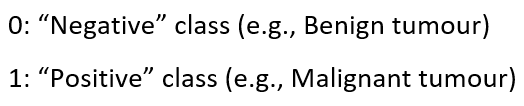This is a classification problem with 2 classes, 0 & 1. Generally, the classification problems have multiple classes say, 0,1,2 and 3.

## Dataset:

### Breast Cancer Wisconsin (Diagnostic) Data Set

Predict whether the cancer is benign or malignant

www.kaggle.com

1. Let’s import the dataset to a pandas dataframe:
``````import pandas as pd
``````

2. The following dataframe is obtained:

``````df.head()

![Image for post](https://miro.medium.com/max/1750/1*PPyiGgocvjHbgIcs9yTWTA.png)

``````

df.info()

### Data analysis:

Let us plot the mean area of the clump and its classification and see if we can find a relation between them.

``````import matplotlib.pyplot as plt
import seaborn as sns
from sklearn import preprocessing
label_encoder = preprocessing.LabelEncoder()
df.diagnosis = label_encoder.fit_transform(df.diagnosis)
sns.set(style = 'whitegrid')
sns.lmplot(x = 'area_mean', y = 'diagnosis', data = df, height = 10, aspect = 1.5, y_jitter = 0.1)
``````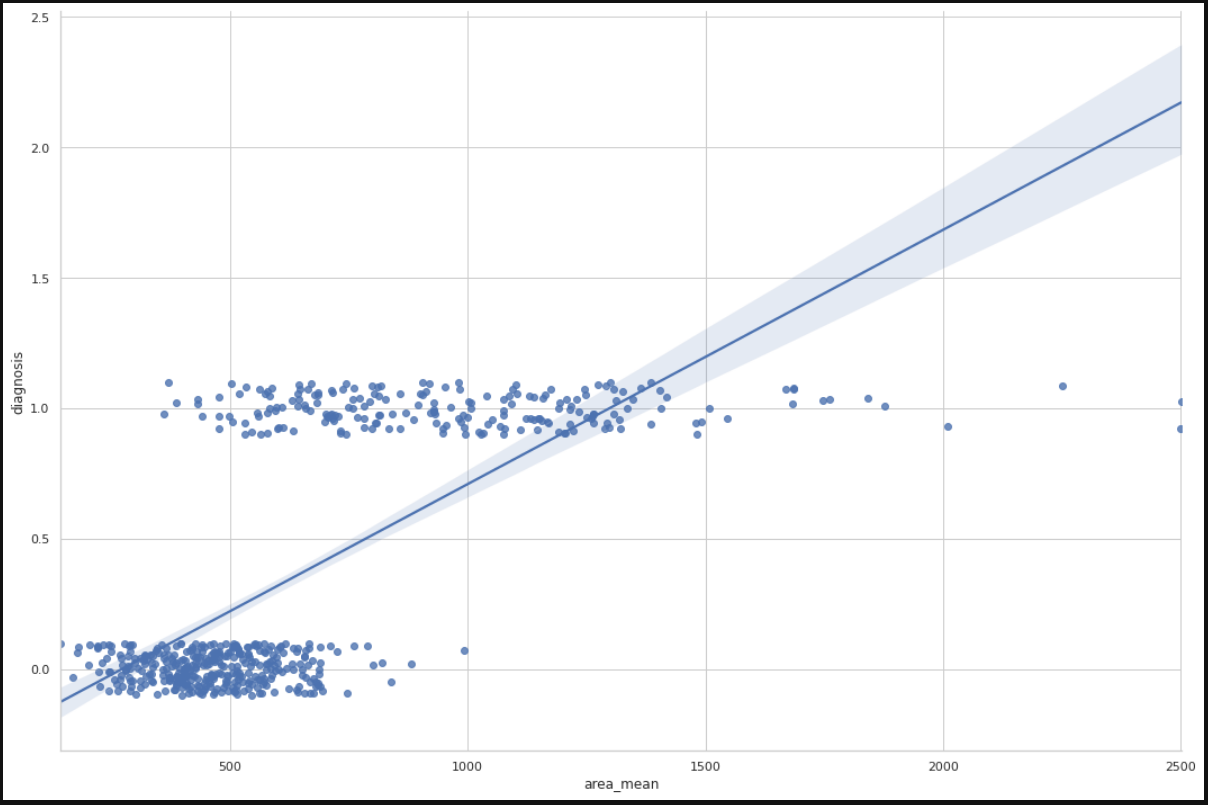We can infer from the plot that most of the tumors having an area less than 500 are benign(represented by zero) and those having area more than 1000 are malignant(represented by 1). The tumors having a mean area between 500 to 1000 are both benign and malignant, therefore show that the classification depends on more factors other than mean area. A linear regression line is also plotted for further analysis.

#machine-learning #logistic-regression #regression #data-sceince #classification #deep learning